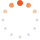# Tistory

티스토리 메뉴 펼치기

티스토리 메뉴 펼치기 댓글수0

프로그래밍/LeetCode

## [LeetCode][Python3] 854. K-Similar Strings

snoopybox
댓글수0

Problem :

https://leetcode.com/problems/k-similar-strings/

My Solution :

class Solution:
def kSimilarity(self, A: str, B: str) -> int:
if A == B:
return 0
checked = set([A])
queue = [A]
ans = 0
while queue:
next_queue = []
for S in queue:
S = list(S)
for i in range(len(S)):
if S[i] != B[i]:
break
for j in range(i+1, len(S)):
if S[j] == B[i] and S[j] != S[i]:
S[i], S[j] = S[j], S[i]
candidate = ''.join(S)
if candidate not in checked:
if candidate == B:
return ans + 1